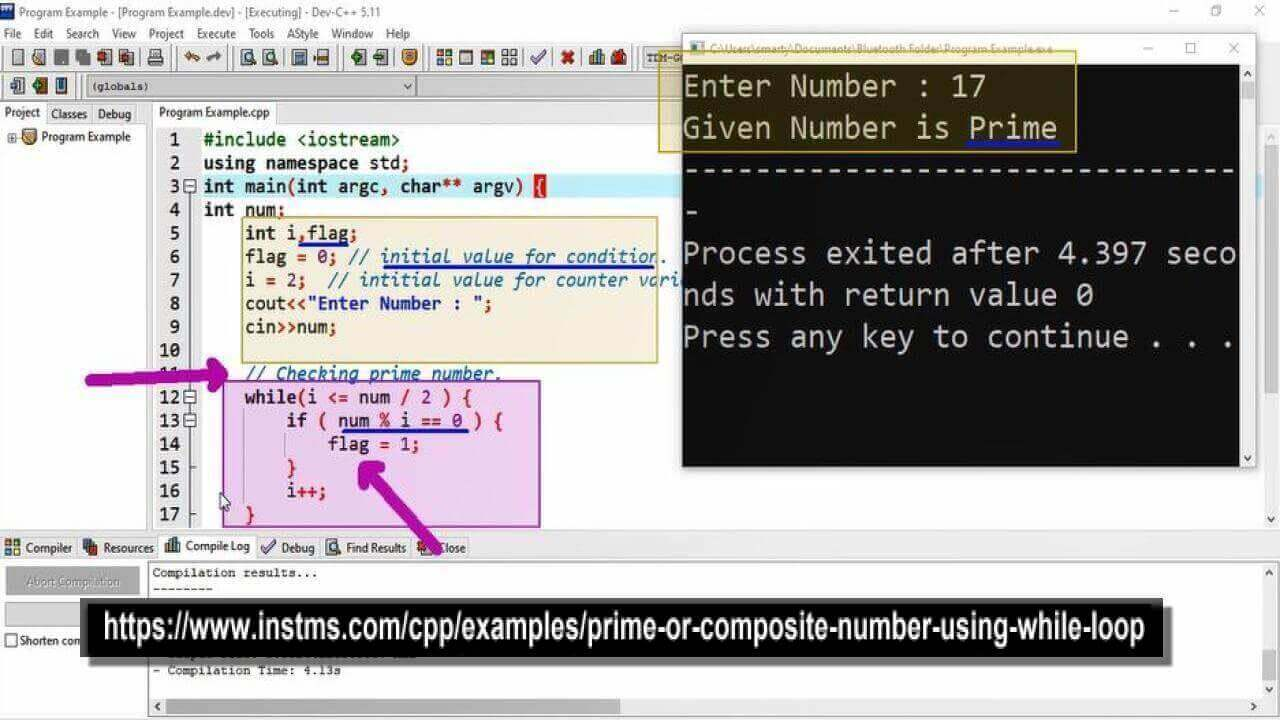# Prime Or Composite Number Using While Loop in CPP

## Source Code

#include <iostream>

using namespace std;

int main(int argc, char** argv) {

int num;
int i,flag;
flag = 0; // initial value for condition.
i = 2;  // intitial value for counter variable.
cout<<"Enter Number : ";
cin>>num;

// Checking prime number.
while(i <= num / 2 ) {
if ( num % i == 0 ) {
flag = 1;
}
i++;
}

if ( flag == 0 ) {
cout<<"Given Number is Prime";
} else {
cout<<"Given Number is Composite";
}
return 0;
}

##Working

Prime numbers are only divisible by one and itself. Let suppose Whe have a numbe N, Then N will call prime only if N % K <> 0 For all K > 1 and K <> N. In this program we declare three variables.

Counter Variable ( To check factors from 2 to given number

Flag Variable (to ensure check for factor of given number)

Num variable (for taking input from user)

In loop iterations we check either any value of counter variable is factor (completly dividing) given number. If counter variable particular value proof as factor of N then we will set flag value as 1 (means change flag set value)..

In last step we will check if flag is changed it means there is factor of given number from 2 to N. Then number will be composite else number will prime.